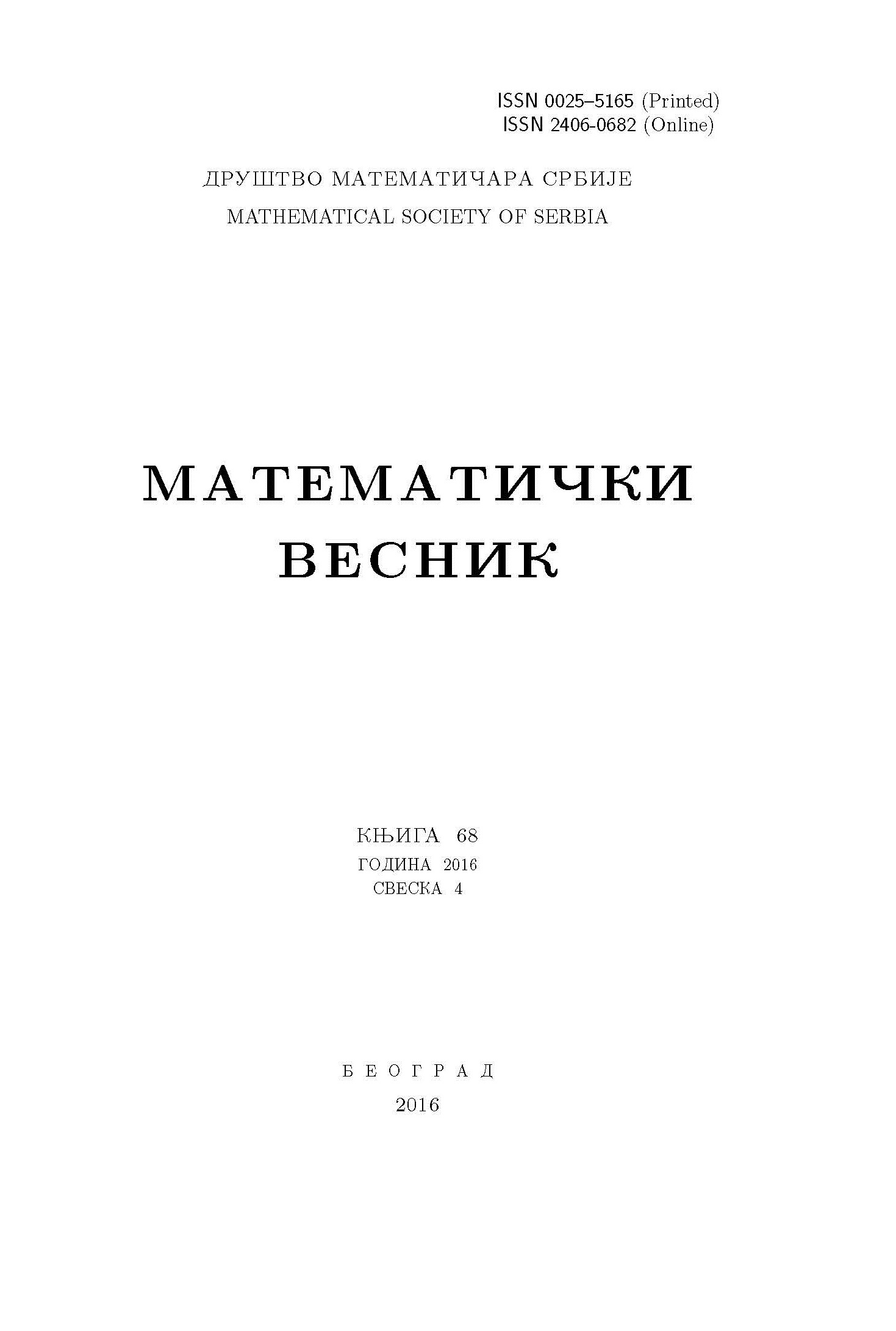﻿ Matematički Vesnik ﻿
 MATEMATIČKI VESNIK МАТЕМАТИЧКИ ВЕСНИКCONSTRUCTION OF UNIVALENT HARMONIC MAPPINGS AND THEIR CONVOLUTIONS C. Singla, S. Gupta, S. Singh AbstractIn this article, we make use of convex analytic functions $H_a(z)=[1/(1-a)]\log[(1-az)/(1-z)]$, $a\in \mathbb{R}$, $|a|\leq 1$, $a\neq 1$ and starlike analytic functions $L_b(z)=z/[(1-bz)(1-z)]$, $b\in \mathbb{R}$, $|b|\leq 1$, to construct univalent harmonic functions by means of a transformation on some normalized univalent analytic functions. Besides exploring mapping properties of harmonic functions so constructed, we establish sufficient conditions for their harmonic convolutions or Hadamard products to be locally univalent and sense preserving, univalent and convex in some direction.Keywords: Harmonic function; univalent function; convolution; convexity in one direction. MSC: 30C45, 30C80 Pages:  1$-$11

﻿